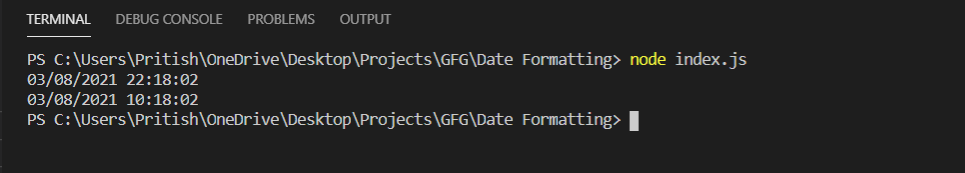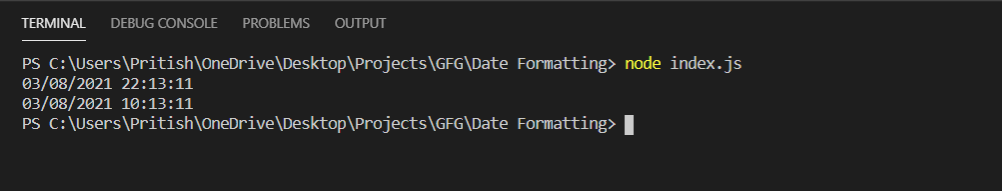How to format the current date in MM/DD/YYYY HH:MM:SS format using Node.js?

• Last Updated : 17 Mar, 2021

The current date can be formatted by using the Nodejs modules like Date Object or libraries like moment.js, dayjs

Method 1: Using Node.js Date Object

The JavaScript Date Object can be used in the program by using the following command.

const date = new Date();

Now on this variable date, we can apply methods to obtain various results according to the requirement. Some methods are:

• getDate(): The method returns the current date.
• getMonth(): The method returns current month.
• getFullYear(): The method returns the current year.
• getHour(): The method returns the current hour in 24-hour format.
• getMinutes(): The method returns minutes of the current hour.
• getSeconds(): The method returns seconds of the current minute.

Below is the implementation of the program:

• In the object format dd is current day, mm is month, yyyy is year, HH is hour in 24-hour format, hh is hour in 12-hour format, MM is minutes, SS is seconds.
• The function formatData() takes an input and checks if it is greater than 9 or not. If it is greater than 10 it will return it without any change but if it is less than 10 then it will append a 0 in front of the input.
• The function formatHour() takes hours as input and converts it according to the 12-hour clock.
• The functions format24Hour() and format12Hour() prints the date in format MM/DD/YYYY HH:MM:SS in 24-hour and 12-hour format respectively.

index.js

 const date = new Date();  // Function to convert// single digit input// to two digitsconst formatData = (input) => {  if (input > 9) {    return input;  } else return `0\${input}`;};  // Function to convert// 24 Hour to 12 Hour clockconst formatHour = (input) => {  if (input > 12) {    return input - 12;  }  return input;};  // Data about dateconst format = {  dd: formatData(date.getDate()),  mm: formatData(date.getMonth() + 1),  yyyy: date.getFullYear(),  HH: formatData(date.getHours()),  hh: formatData(formatHour(date.getHours())),  MM: formatData(date.getMinutes()),  SS: formatData(date.getSeconds()),};const format24Hour = ({ dd, mm, yyyy, HH, MM, SS }) => {  console.log(`\${mm}/\${dd}/\${yyyy} \${HH}:\${MM}:\${SS}`);};const format12Hour = ({ dd, mm, yyyy, hh, MM, SS }) => {  console.log(`\${mm}/\${dd}/\${yyyy} \${hh}:\${MM}:\${SS}`);};  // Time in 24 Hour formatformat24Hour(format);// Time in 12 Hour formatformat12Hour(format);

Run index.js file using below command:

node index.js

Output:Method 2: Using Moment.js Library.

index.js

 const moment = require("moment");  // 24 Hour formatconsole.log(moment().format("MM/DD/YYYY HH:mm:ss"));// 12 Hour formatconsole.log(moment().format("MM/DD/YYYY hh:mm:ss"));

Run index.js file using below command:

node index.js

Output:My Personal Notes arrow_drop_up